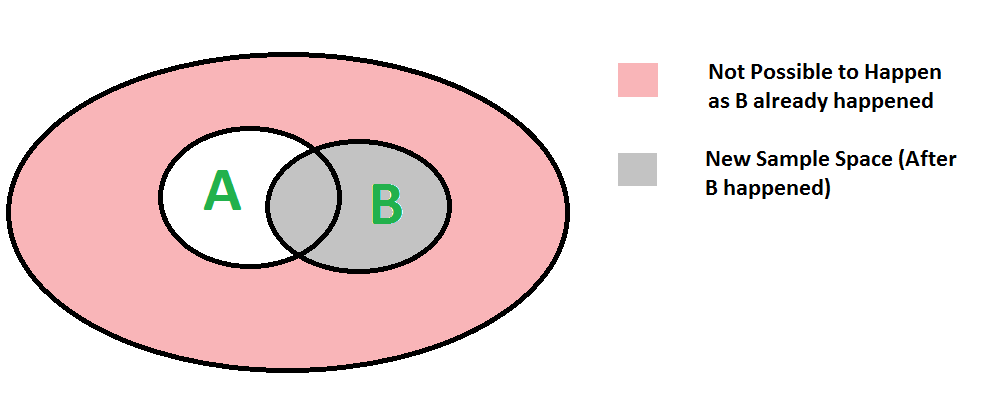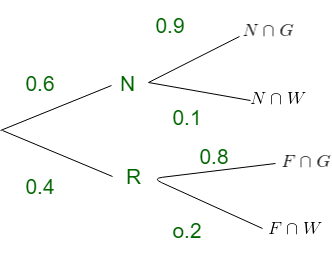Open in App
Not now

# Mathematics | Conditional Probability

• Difficulty Level : Easy
• Last Updated : 28 Jun, 2021

Conditional probability P(A | B) indicates the probability of event ‘A’ happening given that event B happened.We can easily understand the above formula using the below diagram. Since B has already happened, the sample space reduces to B. So the probability of A happening becomesdivided by P(B)Example:
In a batch, there are 80% C programmers, and 40% are Java and C programmers. What is the probability that a C programmer is also Java programmer?

Let A --> Event that a student is Java programmer
B --> Event that a student is C programmer
P(A|B) = P(A ∩ B) / P(B)
= (0.4) / (0.8)
= 0.5
So there are 50% chances that student that knows C also
knows Java 

Product Rule:
Derived from above definition of conditional probability by multiplying both sides with P(B)


P(A ∩ B) = P(B) * P(A|B) 

Understanding Conditional probability through tree:
Computation for Conditional Probability can be done using tree, This method is very handy as well as fast when for many problems.

Example: In a certain library, twenty percent of the fiction books are worn and need replacement. Ten percent of the non-fiction books are worn and need replacement. Forty percent of the library’s books are fiction and sixty percent are non-fiction. What is the probability that a book chosen at random are worn? Draw a tree diagram representing the data.

Solution: Let F represents fiction books and N represents non-fiction books. Let W represents worn books and G represents non-worn books.P(worn)= P(N)*P(W | N) + P(F)*P(W | F)

= 0.6*0.1  +  0.4* 0.2
= 0.14



Exercise:
1) What is the value of P(A|A)?

2) Let P(E) denote the probability of the event E. Given P(A) = 1, P(B) = 1/2, the values of P(A | B) and P(B | A) respectively are (GATE CS 2003)
(A) 1/4, 1/2
(B) 1/2, 1/14
(C) 1/2, 1
(D) 1, 1/2
See this for solution.